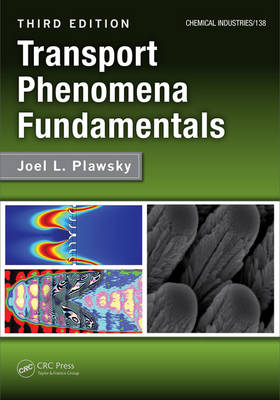•Transport Phenomena Fundamentals - Chemical Industries (Hardback)

(author)
£58.99
Hardback 838 Pages / Published: 23/01/2014
• We can order this

Usually dispatched within 1 week

The third edition of Transport Phenomena Fundamentals continues with its streamlined approach to the subject of transport phenomena, based on a unified treatment of heat, mass, and momentum transport using a balance equation approach. The new edition makes more use of modern tools for working problems, such as COMSOL ®, Maple ®, and MATLAB ®. It introduces new problems at the end of each chapter and sorts them by topic for ease of use. It also presents new concepts to expand the utility of the text beyond chemical engineering.

The text is divided into two parts, which can be used for teaching a two-term course. Part I covers the balance equation in the context of diffusive transport-momentum, energy, mass, and charge. Each chapter adds a term to the balance equation, highlighting that term's effects on the physical behavior of the system and the underlying mathematical description. Chapters familiarize students with modeling and developing mathematical expressions based on the analysis of a control volume, the derivation of the governing differential equations, and the solution to those equations with appropriate boundary conditions.

Part II builds on the diffusive transport balance equation by introducing convective transport terms, focusing on partial, rather than ordinary, differential equations. The text describes paring down the microscopic equations to simplify the models and solve problems, and it introduces macroscopic versions of the balance equations for when the microscopic approach fails or is too cumbersome. The text discusses the momentum, Bernoulli, energy, and species continuity equations, including a brief description of how these equations are applied to heat exchangers, continuous contactors, and chemical reactors. The book also introduces the three fundamental transport coefficients: the friction factor, the heat transfer coefficient, and the mass transfer coefficient in the context of boundary layer theory. The final chapter covers the basics of radiative heat transfer, including concepts such as blackbodies, graybodies, radiation shields, and enclosures. The third edition incorporates many changes to the material and includes updated discussions and examples and more than 70 new homework problems.

Publisher: Taylor & Francis Inc
ISBN: 9781466555334
Number of pages: 838
Weight: 1882 g
Dimensions: 254 x 178 x 51 mm
Edition: 3rd New edition